# Algebra 1 : How to find mode

## Example Questions

### Example Question #151 : How To Find Mode

A test was given to a class ofstudents. Three students scored, three scored, three scoredand two scored. What was the modal score (mode) for this test for this class?There is no mode for this set.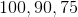Explanation:is definitely incorrect because it occurs only twice while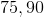andoccur thrice. Sinceoccur thrice and mode is the data point that repeats the most, henceare all modes or modal scores.

### Example Question #152 : How To Find Mode

Find the mode of the data set: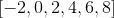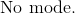Explanation:

The mode is defined as the highest frequency of a number or numbers in a data set.

Each number in the given set has a frequency of one.

This means that there is no mode.

The answer is:### Example Question #152 : How To Find Mode

Find the mode of the data set: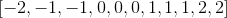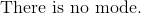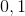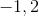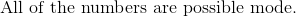Explanation:

The mode is defined as the highest occurrence of numbers in a given set of data.

The numbers that occur three times in the given set of data are:The numbers that occur twice in the data set are not the highest occurrence of numbers.

The answer is:### Example Question #154 : How To Find Mode

Find the mode: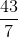Explanation:

The mode is the number or numbers that has the highest occurrence in a dataset.

The only number that occurs more than once is seven.

Every other number in the given set of data only has an occurrence of one.

The answer is:### Example Question #151 : How To Find Mode

Find the mode of the data set: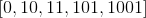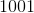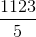Explanation:

The mode is the highest frequency of numbers that occur set of data.

Do not count individual ones, tens, and hundreds digits as the frequency of occurrence.

Since each number occurs only once, there is no mode.

The answer is:### Example Question #151 : How To Find Mode

Find the mode of the set of data: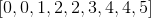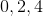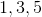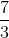Explanation:

The mode is defined as the highest frequency of number or numbers in the given set of data.

The highest frequency of numbers are zero, two, and four, since they appear twice in the set.

The answer is:### Example Question #157 : How To Find Mode

The test scores for a science test are as follows: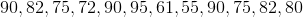What is the mode?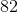Explanation:

The mode is the number that occurs most often in a data set.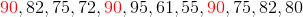Because 90 appears three times--and no other number occurs as many times--90 is the mode.

### Example Question #151 : How To Find Mode

The batting averages of the starting lineup for a baseball team are as follows: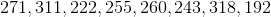What is the mode?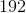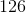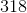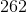None

None

Explanation:

The mode is the number that occurs most often in a data set.Because each batting average occurs only once, there is no mode.

### Example Question #151 : How To Find Mode

The average daily temperatures for the last week in Miami are as follows: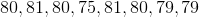What is the mode?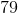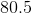Explanation:

The mode is the number that occurs most often in a data set.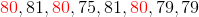Because 80 appears three times and each other temperature occurs only once or twice, 80 is the mode.

### Example Question #521 : Statistics And Probability

The favorite numbers of a class of students are as follows: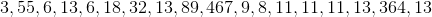What is the mode?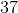Explanation:

The mode is the number that occurs most often in a data set.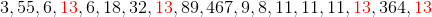Because 13 appears four times and each other number occurs once, twice, or three times, 13 is the mode.

### All Algebra 1 Resources